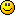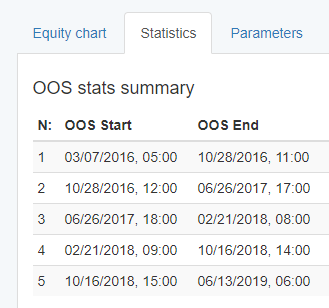# forex software

Create and Test Forex Strategies

forex software

Forex Software

Create and Test Forex Strategies

# Walk Forward Analysis - calculations of the segments' length.

Forex Software → Walk Forward Analysis - calculations of the segments' length.

Pages 1

## Posts: 9

#### Topic: Walk Forward Analysis - calculations of the segments' length.

Hi
An error was detected during testing: there are some extra days beetween start/end segment datesAlso why the period OOS is different from the data in the table#### Re: Walk Forward Analysis - calculations of the segments' length.

Yes as for holidays - clear now.
But there is the first question - sometimes there is extra days during WFA (i can't understand logic of that and how it affect on quality of testing)#### Re: Walk Forward Analysis - calculations of the segments' length.

The Walk Forward formulas look right. It calculates the segments' length without the necessary bars for initializing the strategy.

Let's say you have 20 000 bars and your strategy uses 100 bars for initialization. It means that the Walk Forward tool will use 19900 bars. Let's have 5 segments and 30% OOS. The formula for calculating the segment's bars is:

``segmentBars = 100 * (bars - firstBar) / ((100 - oosPercent) + segments * oosPercent);``

For the above number, we will have segment bars = 100 * 19900 / ((100 - 30) + 5 * 30) = 1990000 / (70 + 150) = 9045 bars.

We will split these 9045 bars to In Sample part and OOS part as follows:

OOS bars = segmentBars * oosPercent / 100 = 9045 * 30 / 100 = 2714 bars.
IS bars = segmentBars - OOS bars = 9045 - 2714 = 6331 bars.

So we have:
Segment 1 starts at bar 100 + 0 * 2714 = 100   and ends at bar 100 + 9045 = 9145.
Segment 2 starts at bar 100 + 1 * 2714 = 2814 and ends at bar 2814 + 9045 = 11859.
...
Segment 5 starts at bar 100 + 4 * 2714 = 10956 and ends at bar 10956 + 9045 = 20001

You see everithing is fine. We have 1 bar extrabecause I round the calculations in this example.
Each segment walks forward with exactly OOS bars (2714).

After this math, the program takes the exact date from the corresponding bar.

Walk forward calculates the count of days as follows: bars * period / 1440

If it is M15 chart, it will be:

In Sample days = 6331 * 15 / 1440 = 66 days.
Out of Sample days = 2714 * 15 / 1440 = 28 days.

I would be glad to help if anything is not clear.

#### Re: Walk Forward Analysis - calculations of the segments' length.

Ooo I see.

The table columns titles are not correct. The table shows the OOS stats only and the columns must be OOS Start and  OOS End

#### Re: Walk Forward Analysis - calculations of the segments' length.

Now is better.

Each OOS period starts exactly at the following bar of the end of the previous OOS period.#### Re: Walk Forward Analysis - calculations of the segments' length.

Miroslav, has logic of WFA changed from yesterday?
Because systems that have passed WFA yesterday does not pass it now (option are the same)

#### Re: Walk Forward Analysis - calculations of the segments' length.

The logic is the same. There are more options to set now.

I'll make further improvement of the validation next days.

#### Re: Walk Forward Analysis - calculations of the segments' length.

Popov wrote:

The logic is the same. There are more options to set now.

I'll make further improvement of the validation next days.

Could you test one of yours systems and check that result is the same as it was?

I test using WFA (in Chrome and IE) and every my systems and all results are differ from what it was.

Pages 1